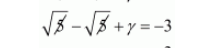# If 5–√ and −5–√ are two zeroes of the polynomial x3 + 3x2 − 5x − 15,`
Question:

If $\sqrt{5}$ and $-\sqrt{5}$ are two zeroes of the polynomial $x^{3}+3 x^{2}-5 x-15$, then its third zero is

(a) 3
(b) −3
(c) 5
(d) −5

Solution:

Let $\alpha=\sqrt{5}$ and $\beta=-\sqrt{5}$ be the given zeros and $\gamma$ be the third zero of the polynomial $x^{3}+3 x^{2}-5 x-15$. Then,

By using $\alpha+\beta+\gamma=\frac{-\text { Coefficient of } x^{2}}{\text { Coefficient of } x^{3}}$

$\alpha+\beta+\gamma=\frac{-3}{1}$

$\alpha+\beta+\gamma=-3$

Substituting $\alpha=\sqrt{5}$ and $\beta=-\sqrt{5}$ in $\alpha+\beta+\gamma=-3$

We get

$\sqrt{5}-\sqrt{5}+\gamma=-3$$\gamma=-3$

Hence, the correct choice is $(b)$